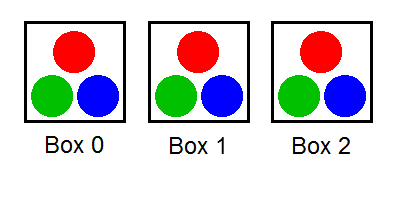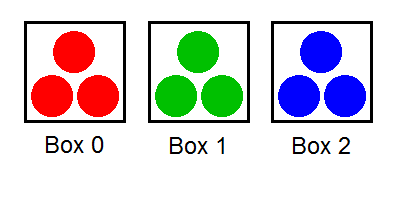## TopCoder SRM 568 BallsSeparating 作者是 在线疯狂 发布于 2014年5月10日 在 topcoder-srm.

Topcoder Single Round Match 568

Problem Statement
There are N boxes numbered from 0 to N-1, inclusive. For each i, box i contains red[i] red balls, green[i] green balls, and blue[i] blue balls.
Fox Ciel wants to separate the balls by colors. In each operation, she can pick a single ball from some box and put it into another box. She considers the balls to be separated if no box contains balls of more than one color.
Return the minimal number of operations required to separate the balls. If this is impossible, return -1.

Definition

Class: BallsSeparating
Method: minOperations
Parameters: int[], int[], int[]
Returns: int
Method signature: int minOperations(int[] red, int[] green, int[] blue)
(be sure your method is public)

Constraints
- red, green and blue will each contain between 1 and 50 elements, inclusive.
- red, green and blue will contain the same number of elements.
- Each element of red, green and blue will be between 1 and 1,000,000, inclusive.'

Java代码

``````
public class BallsSeparating {

public static void main(String[] args) {
BallsSeparating bs = new BallsSeparating();
int[] red = {842398, 491273, 958925, 849859, 771363, 67803, 184892, 391907, 256150, 75799};
int[] green = {268944, 342402, 894352, 228640, 903885, 908656, 414271, 292588, 852057, 889141};
int[] blue = {662939, 340220, 600081, 390298, 376707, 372199, 435097, 40266, 145590, 505103};

int ans = bs.minOperations(red, green, blue);
System.out.println(ans);
}

public int minOperations(int[] red, int[] green, int[] blue) {
int boxNum = red.length;
if (boxNum < 3) {
return -1;
}

int dp[][] = new int[boxNum + 1];
for (int i = 0; i < 8; i++) {
for (int j = 0; j <= boxNum; j++) {
dp[i][j] = -1;
}
}
dp = 0;
for (int i = 1; i <= boxNum; i++) {
int r = red[i - 1];
int g = green[i - 1];
int b = blue[i - 1];
for (int k = 0; k < 3; k++) {
for (int j = 0; j < 8; j++) {

//BGR
int code = getCode(j,k);
int val = getValue(r,g,b,k);
if (dp[j][i - 1] >= 0) {
if (dp[code][i] < 0)
dp[code][i] = dp[j][i - 1] + val;
else
dp[code][i] = Math.min(dp[code][i], dp[j][i - 1] + val);
}
System.out.print(dp[code][i] + " ");
}
System.out.println();
}
System.out.println();
}
return dp[boxNum];
}

public int getValue(int r, int g, int b, int color) {
if (color == 0) //B
return r + g;
else if (color == 1) //G
return r + b;
else //R
return g + b;
}
//210
//RGB
public int getCode(int curCode, int color) {
return curCode | (1 << color);
}
}
``````Pingbacks已关闭。